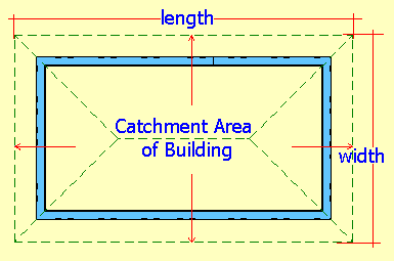Spike's Calculators

# Collecting Rain Water from a Catchment Area/ Inch per Square Foot

The catchment area is the area from which rainfall is collected. If you are using the roof of a building for this purpose, calculate the square footage of this area by taking the length of the building, including the overhang(s) and multiply this by the width measurement plus the overhang(s) of the building.##### Example:
Rainfall = 2" per square foot
square footage of catchment area = 2000 sq ft
you won't be able to collect 100% of the water
this catchment area is 80% efficient, meaning you will lose 20% of the water
the amount of rain and the square footage has to be in the same measurements.
`convert the area in square feet to square inches to be able to do the calculation`
converting square footage to square inches: there are 144 sq inches in one square foot

2000 * 144 = 288000 sq inches = 288000 * 2 = 576000 cubic inches of water. There are 231 cubic inches in one US gallon -- 576000 / 231 = 2494 US gallons of water @ 100% efficiency @ 80% -- 2494 * (80 ÷ 100) = 1995.2 US gallons of water @ 80% efficiency

### Rainfall/Inch per Square Foot

Catchment Area Square Footage ft²
Rainfall Inch per Square Foot in/ft²
Efficiency of Collecting the Water %

#### Results:

 Cubic Inches in³ US Gallons US gal Imperial Gallons Imp gal Litres L

#### Calculation

1. rainfall amount, measured in inches per square foot
2. total square footage of the catchment area
3. efficiency (preset @ 80%)

#### Results

the amount of water collected from the catchment area in
1. cubic inches
2. US gallons
3. Imperial gallons
4. litres